12

1. The examination will comprise of  Single Correct Objective type Multiple Choice Questions (MCQs)
2. All questions are compulsory and each carries Four marks.
3. The total number of questions and duration of the examination are displayed on the navigation panel.
4. There will be NO NEGATIVE MARKING for the wrong answers.
5. The students just need to click on the Right Choice / Correct option from the multiple choices /options given with each question. For Multiple Choice Questions, each question has four options, and the candidate has to click the appropriate option.
6. The Time of the examination begins only when the ‘Start Test’ button is pressed.
7. The answers can be changed at any time during the test and are saved automatically.
8. The examinee can move to First, Last, Previous, Next, and unanswered questions by clicking on the buttons with respective labels displayed on the screen throughout the test
9. The Time remaining is shown in the middle of the screen.
10. The system automatically shuts down when the time limit is over OR alternatively if the examinee finishes the exam before the time he can quit by pressing the ‘FINISH Test’ button. The students don’t click the “FINISH TEST” Button until the student wants to quit from Examination.
11. Final marks will be displayed just after completion of the test.

1 / 35

Que: A number x is chosen at random from the numbers -3, -2, -1, 0, 1, 2, 3 the probability  that  is

2 / 35

Que: An element X is soft and can be cut with a knife. This is very reactive to air and cannot be kept open in air. It reacts vigorously with water. Identify the element from the following

1. Mg
2. Na
3. P
4. Ca

3 / 35

Que: Which of the following statements is correct about an aqueous solution of an acid and of a base?

(i) Higher the pH, stronger the acid
(ii) Higher the pH, weaker the acid
(iii) Lower the pH, stronger the base
(iv) Lower the pH, weaker the base

1. (i) and (iii)
2. (ii) and (iii)
3. (i) and (iv)
4. (ii) and (iv)

4 / 35

Que: One kWh is equal to

1. 3.6 × 106 MJ
2. 3.6 × 105 MJ
3. 3.6 × 10² MJ
4. 3.6 MJ

5 / 35

Que: If  the sum of  the areas of  two circles with radii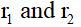is equal to the  area of a circle of radius  r, then

1. r = r1 + r2
2.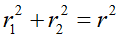3. r1 + r2 < r
4.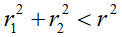6 / 35

Que: What is that instrument which can detect the presence of electric current in a circuit?

1. galvanometer
2. motor
3. generator
4. none of above

7 / 35

Que: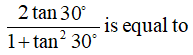1. sin
2. cos
3. tan
4. sin

8 / 35

Que: Look at this series: 1.5, 2.3, 3.1, 3.9, ... What number should come next?

1. 4.2

2. 4.4

3. 4.7

4. 5.1

9 / 35

Que: If  the area of a sector of a circle is of the area of the circle, then the sector angle is equal to

10 / 35

Que: A number is selected from the first 50 natural numbers. What is the probability that it is a multiple of 3 or 5?

11 / 35

Que: Under which of the following conditions can a concave mirror form a Real image larger than the actual object?

1. When the object is kept at a distance equal to its radius of curvature
2. When object is kept at a distance less than its focal length
3. When object is placed between the focus and centre of curvature
4. When object is kept at a distance greater than its radius of curvature

12 / 35

Que: If the system of equations 2x + 3y = 7 and, (a + b)x+ (2a - b)y = 21 has infinitely many solutions, then

1. a = 1, b = 5
2. a = 5, b = 1
3. a = -1, b = 5
4. a = 5, b = -1

13 / 35

Que: The process in which a carbonate ore is heated strongly in the absence of air to convert it into metal oxide is called

1. Roasting
2. Reduction
3. Calcination
4. Smelting

14 / 35

Que: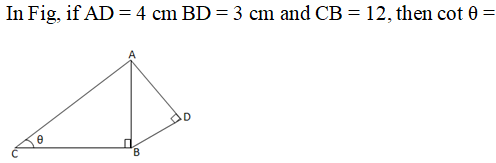15 / 35

Que: Assertion: Ammonium hydroxide is Weak Base

Reason: Phenolphthalein becomes pink in NH2OH

1. Both A and R are true and R is the correct explanation of A.
2. Both A and R are true but R is not the correct explanation of A.
3. A is true but R is false.
4. A is false but R is true.

16 / 35

Que: The amount of Heat Energy Produced in 5 Minutes by an electric heater having a power rating of 1000 watts.

1. 200,000 J
2. 300,000 J
3. 400,000 J
4. 300 J

17 / 35

Que: If a = and LCM (a, b, c) = then n =

1. One
2. Two
3. Three
4. Four

18 / 35

Que: A light ray enters from medium A to medium B as shown in the figure. The refractive index of medium B relative to A will be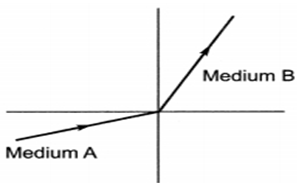1. greater than unity
2.  less than unity
3. equal to unity
4. zero

19 / 35

Que: Copper objects lose their shine and form a green coating of

1. Copper oxide
2. Copper hydroxide and Copper oxide
3. Basic Copper carbonate
4. Copper carbonate

20 / 35

Que: An electric iron draws a current 4 A when connected to a 220 V mains. Its resistance must be

1. 1000 Ω
2. 55 Ω
3. 44 Ω
4. None of these

21 / 35

Que: A person cannot see distinctly objects kept beyond 2 m. This defect can be corrected by using lens of power

1. +0.5 D
2. -0.5 D
3. -0.5 D
4. -0.2 D

22 / 35

Que: The ratio in which (4, 5) divides the lines segment joining the points (2, 3) and (7, 8) is

1. -2 : 3
2. -3: 2
3. 3: 2
4. 2 : 3

23 / 35

Que: Electrolysis of water is a decomposition reaction. The mole ratio of oxygen and hydrogen gases liberated during electrolysis of water is:

1. 1: 1
2. 2: 1
3. 4: 1
4. 1: 2

24 / 35

Que: Look at this series: 201, 202, 204, 207, ... What number should come next?

1. 205

2. 208

3. 210

4. 211

25 / 35

Que: Which of the given statements is not a physical change?

1. Boiling of water to give water vapour
2. Melting of ice to give water
3. Dissolution of salt in water
4. Combustion of Liquefied Petroleum Gas (LPG)

26 / 35

Que: Which of the given shapes would complete the sequence?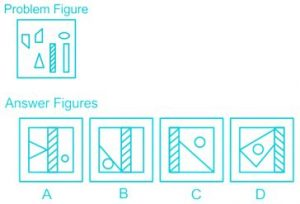1. D

2. B

3. C

4. A

27 / 35

Que: A student sitting on the last bench can read the letters written on the blackboard but is not able to read the letters written in his textbook. Which of the following statements is correct?

1. The near point of his eyes has receded away.
2. The near point of his eyes has come closer to him
3. The far point of his eyes has come closer to him
4. The far point of his eyes has receded away

28 / 35

Que: Which of the following is an endothermic process?

1. Dilution of sulphuric acid
2. Sublimation of dry ice
3. Condensation of water vapours
4. Respiration in human beings

29 / 35

Que: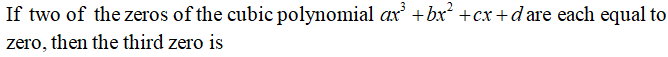30 / 35

Que: Rahul put his timepiece on the table in such a way that at 6 P.M. hour hand points to North. In which direction the minute hand will point at 9.15 P.M. ?

1. South-East

2. South

3. North

4. West

31 / 35

Que: An aqueous solution turns red litmus solution blue. Excess addition of which of the following solution would reverse the change?

1. Baking powder
2. Lime
3. Ammonium hydroxide solution
4. Hydrochloric acid

32 / 35

Que: The position of how many digits in the number 2319763518945 shall remain the same if the digits are arranged in ascending order?

1. One

2. Three

3. Six

4. Five

5. Three

33 / 35

Que: What is the oxidation number of chlorine in HClO4?

1. +3
2. +5
3. +7
4. +8

34 / 35

Que: The power of the lens is -4, its focal length is

1. 4m
2. -40m
3. -0.25m
4. -25m

35 / 35

Que: A concave mirror gives real, inverted, and same size images if the object is placed

1. At F
2. At infinity
3. At C
4. Beyond C